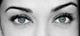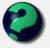# Math Is Fun Forum

Discussion about math, puzzles, games and fun.   Useful symbols: ÷ × ½ √ ∞ ≠ ≤ ≥ ≈ ⇒ ± ∈ Δ θ ∴ ∑ ∫  π  -¹ ² ³ °

You are not logged in.

## #1 2006-11-22 04:40:44

unique
MemberRegistered: 2006-10-04
Posts: 419

### central angle

the central angle of a sector of a circle is 0.6 radian.
find the area of the sector if the radius is 4 meters.

i did:

0.6 = 0.6 / 360 * pi(4)^2 = 0.026666666

correct?

Desi
Raat Key Rani !

Offline

## #2 2006-11-22 06:03:56

mathsyperson
ModeratorRegistered: 2005-06-22
Posts: 4,900

### Re: central angle

Almost. You would be correct if the angle of the sector was 0.6 degrees, but it's actually 0.6 radians.

That means that instead of dividing by 360, you divide by 2π, which actually makes the working out a bit easier.

Area = 0.6/2π * π*4² = 0.6/2 * 16 = 0.48m².

Why did the vector cross the road?
It wanted to be normal.

Offline